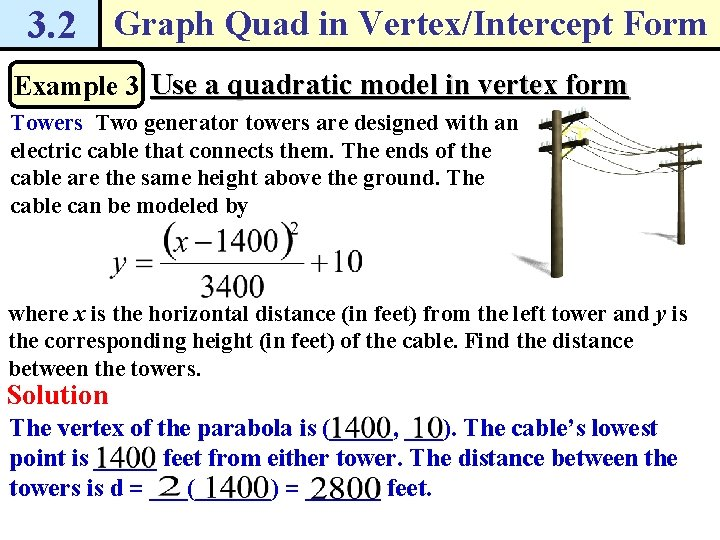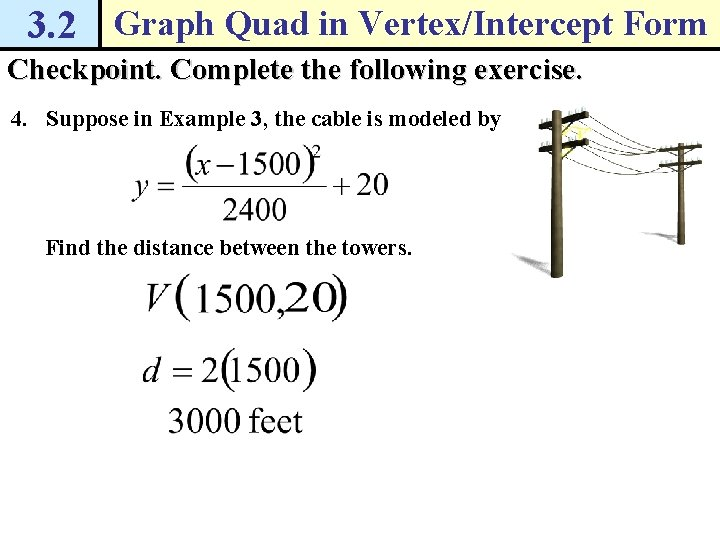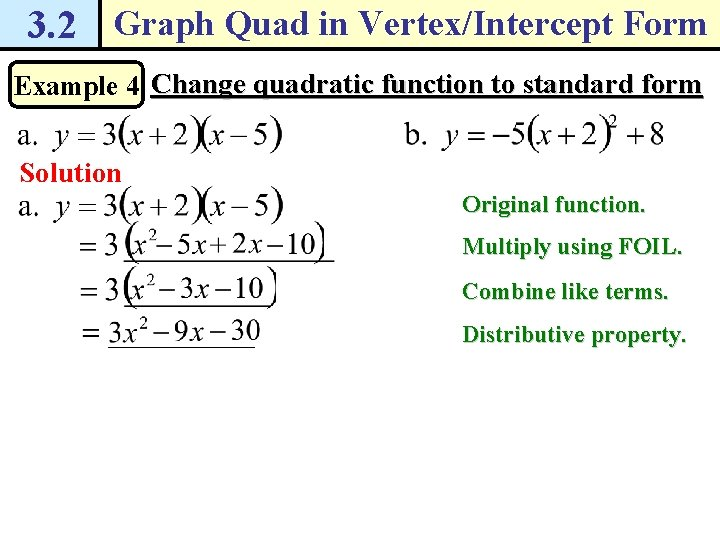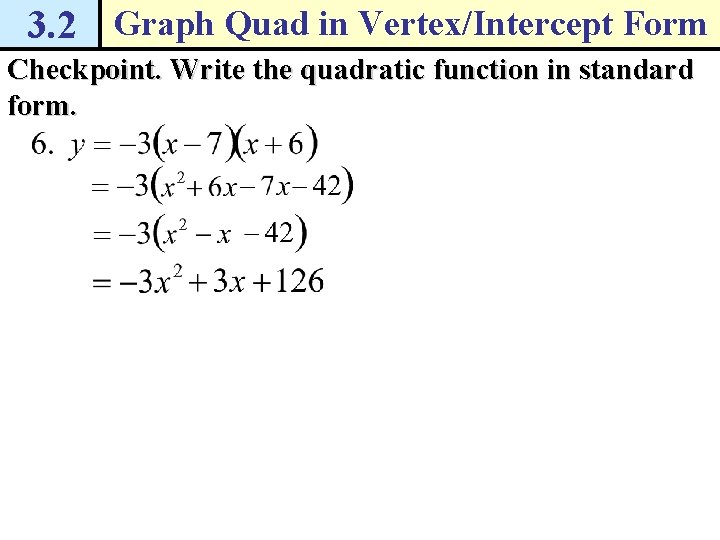# How do I graph quadratic functions in vertex

• Slides: 15• How do I graph quadratic functions in vertex and intercept form? • How do I rewrite a quadratic in standard form?3. 2 Graph Quad in Vertex/Intercept Form Example 1 Graph a quadratic function in vertex form Graph Solution 1. Identify the constants a = ____, h = ___, and k = ____. Because a > 0, the parabola opens ____. 2. Plot the vertex (h, k) = (___, ___) and draw the axis of symmetry at x = ____. 3. Use the pattern for the parabola to plot the other points. a = ____, so the graph is going up half as fast as normal. 4. Draw a parabola through the plotted points.3. 2 Graph Quad in Vertex/Intercept Form Checkpoint. Graph the function. Label the vertex and axis of symmetry.3. 2 Graph Quad in Vertex/Intercept Form Example 2 Graph a quadratic function in intercept form Graph Solution 1. Identify the x-intercepts. Because p = ____ and q = ____, the x-intercepts occur at the points (___, 0) and (___, 0). 2. Find the coordinates of the vertex. So, the vertex is (___, ___). 4. Draw a parabola through the vertex and the points where the x-intercepts occur.3. 2 Graph Quad in Vertex/Intercept Form Checkpoint. Graph the function. Label the vertex, axis of symmetry, and x-intercepts.3. 2 Graph Quad in Vertex/Intercept Form Checkpoint. Graph the function. Label the vertex, axis of symmetry, and x-intercepts.3. 2 Graph Quad in Vertex/Intercept Form Example 3 Use a quadratic model in vertex form Towers Two generator towers are designed with an electric cable that connects them. The ends of the cable are the same height above the ground. The cable can be modeled by where x is the horizontal distance (in feet) from the left tower and y is the corresponding height (in feet) of the cable. Find the distance between the towers. Solution The vertex of the parabola is (_____, ___). The cable’s lowest point is _____ feet from either tower. The distance between the towers is d = ___(______) = ______ feet.3. 2 Graph Quad in Vertex/Intercept Form Checkpoint. Complete the following exercise. 4. Suppose in Example 3, the cable is modeled by Find the distance between the towers.3. 2 Graph Quad in Vertex/Intercept Form Example 4 Change quadratic function to standard form Solution Original function. Multiply using FOIL. Combine like terms. Distributive property.3. 2 Graph Quad in Vertex/Intercept Form Example 4 Change quadratic function to standard form Solution Original function. Rewrite (x + 2)2. Multiply using FOIL. Combine like terms. Distributive property. Combine like terms.3. 2 Graph Quad in Vertex/Intercept Form Checkpoint. Write the quadratic function in standard form.3. 2 Graph Quad in Vertex/Intercept Form Checkpoint. Write the quadratic function in standard form.3. 2 Graph Quad in Vertex/Intercept Form Pg. 67, 3. 2 #1 -29